Updating search results...

# 50 Results

View
Selected filters:
• NC.M1.G-GPE.4Rating
0.0 stars

Students learn how to use the Pythagorean theorem and are introduced to an important application of it.

Subject:
Mathematics
Material Type:
Activity/Lab
Provider:
University of Kentucky
Author:
Julie Gibbs and Tara Bennett
02/26/2019Rating
0.0 stars

Practice and explanation of using distance formula to calculate area and perimeter of shapes in the coordinate plane.

Subject:
Mathematics
Material Type:
Activity/Lab
Provider:
Math Worksheets Land
02/13/2018Rating
0.0 stars

In this Khan Academy activity, students find the area and perimeter of a shape on a coordinate plane.

Subject:
Mathematics
Material Type:
Activity/Lab
Provider:
08/23/2018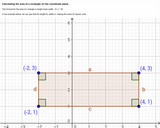Unrestricted Use
CC BY
Rating
0.0 stars

Images of rectangle on coordinate plane, one with easily identifiable area, other image with same rectangle rotated. Distance formula shown to calculate same area.

Subject:
Geometry
Mathematics
Material Type:
Interactive
Provider:
Michigan Virtual
07/19/2019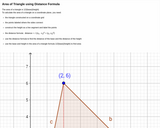Unrestricted Use
CC BY
Rating
0.0 stars

Definition of area of triangle, image of triangle on coordinate grid with height constructed. Area of triangle calculated using distance formula.

Subject:
Geometry
Mathematics
Material Type:
Interactive
Provider:
Michigan Virtual
07/19/2019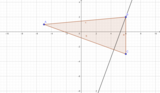Unrestricted Use
CC BY
Rating
0.0 stars

This activity promotes critical thinking and experimenting with triangles.

Subject:
Mathematics
Material Type:
Activity/Lab
Author:
VIJAYALAKSHMI SANKARAN
05/27/2020Conditional Remix & Share Permitted
CC BY-NC-SA
Rating
0.0 stars

This task explores the real world topic of building light rails. Throughout the implementation of this task the students will learn about the cost of building railways and how to implement them within a budget. This task explores such mathematical concepts of using coordinates to find the distance between points, using coordinates to build polygons and find the area and length of sides, and writing equations of parallel lines.

Subject:
Algebra
Geometry
Material Type:
Activity/Lab
Author:
Carrie Robledo
James O'Neal
03/26/2021Conditional Remix & Share Permitted
CC BY-NC-SA
Rating
0.0 stars

CK-12 Foundation's Geometry FlexBook is a clear presentation of the essentials of geometry for the high school student. Topics include: Proof, Congruent Triangles, Quadrilaterals, Similarity, Perimeter & Area, Volume, and Transformations.

Subject:
Mathematics
Material Type:
Textbook
Provider:
CK-12 Foundation
Provider Set:
CK-12 FlexBook
10/06/2009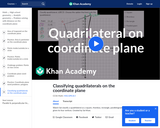Rating
0.0 stars

This video classifies shapes as quadrilaterals based on slope.

Subject:
Mathematics
Material Type:
Demonstration
Provider:
02/28/2018Rating
0.0 stars

Lesson and practice problems using midpoint, slope and distance formula to prove that figures are certain types of quadrilaterals.

Subject:
Mathematics
Material Type:
Activity/Lab
Provider:
Miami Arts Charter School
02/19/2018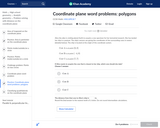Rating
0.0 stars

In this Khan Academy activity, students will solve word problems on a coordinate plane using the formulas for distance, area, and perimeter.

Subject:
Mathematics
Material Type:
Activity/Lab
Provider:
08/23/2018Rating
0.0 stars

Class activity using distance formula, midpoint formula and cost to plan out a fictional trip

Subject:
Mathematics
Material Type:
Activity/Lab
Provider:
Weebly
11/10/2017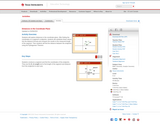Rating
0.0 stars

Texas Instruments activity exploring distances in the coordinate plane in order to classify shapes.

Subject:
Mathematics
Material Type:
Activity/Lab
Provider:
Texas Instruments
03/07/2018Conditional Remix & Share Permitted
CC BY-NC
Rating
0.0 stars

Students will use distance and perimeter on the coordinate plane to determine which fence company offers the best price for fencing a yard. Depending on the level of the class, students can use the Pythagorean Theorem or the distance formula.

Subject:
Mathematics
Material Type:
Activity/Lab
Assessment
Formative Assessment
Homework/Assignment
07/10/2020Conditional Remix & Share Permitted
CC BY-NC
Rating
0.0 stars

Students will use distance and perimeter on the coordinate plane to determine which fence company offers the best price for fencing a yard. Depending on the level of the class, students can use the Pythagorean Theorem or the distance formula.

Subject:
Mathematics
Material Type:
Activity/Lab
Assessment
Formative Assessment
Homework/Assignment
06/08/2020Conditional Remix & Share Permitted
CC BY-NC
Rating
0.0 stars

Students will use distance and perimeter on the coordinate plane to determine which fence company offers the best price for fencing a yard. Depending on the level of the class, students can use the Pythagorean Theorem or the distance formula.

Subject:
Mathematics
Material Type:
Activity/Lab
Assessment
Formative Assessment
Homework/Assignment
11/06/2019Conditional Remix & Share Permitted
CC BY-NC
Rating
0.0 stars

Students will use distance and perimeter on the coordinate place to determine which fence company offers the best price for fencing a yard. Depending on the level of the class, students can use the Pythagorean Theorem or the distance formula.

Subject:
Mathematics
Material Type:
Activity/Lab
Assessment
Formative Assessment
Homework/Assignment
11/06/2019Rating
0.0 stars

Editable PPT explaining the distance formula and application of it.

Subject:
Mathematics
Material Type:
Presentation
Provider:
serratellimath.wordpress.com
07/06/2018Conditional Remix & Share Permitted
CC BY-NC-SA
Rating
0.0 stars

CK-12's Basic Geometry FlexBook is designed to present students with geometric principles in a simpler, more graphics-oriented course. Students will explore geometry at a slower pace with an emphasis placed on visual aids and approachability.

Subject:
Mathematics
Material Type:
Textbook
Provider:
CK-12 Foundation
Provider Set:
CK-12 FlexBook
Author:
Cifarelli, Victor
Farbizio, Annamaria (Editor)
Gloag, Andrew
Greenberg, Dan
Jordan, Lori
Sconyers, Jim
Zahner, Bill
10/11/2010Conditional Remix & Share Permitted
CC BY-NC-SA
Rating
0.0 stars

Given a physical situation (e.g., a room of a certain shape and dimensions, with objects at certain positions and a robot moving across the room), students impose a coordinate system and describe the given in terms of polygonal regions, line segments, and points in the coordinate system.

Subject:
Math 1
Mathematics
Material Type:
Lesson
Author:
EngageNY